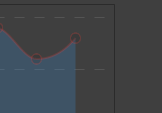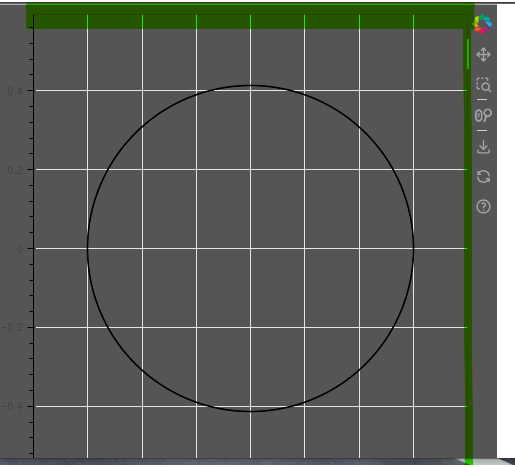# Figure - Outline alpha

Hello all,

I wanted to make consistant background look of my charts and found that Im not able to make it.

Figure has few basic tools:

1. Backgroud
2. Outline
3. Border
4. is there something I miss? → I cannot get rid of the line(marked by green in example)

If I use Background.alpha, and Outline.alpha charts has not same look forhow to make “Outline” share same paramets as background not to look different?

Simplified example:``````from bokeh.plotting import figure, show
from math import pi, sin, cos

plot = figure(title=None, width=500, height=500, x_range = [-4,4], match_aspect=True)

# BackGround Colors
plot.background_fill_color = "#2A2A2A"
plot.background_fill_alpha = 0.8

# Outline
plot.outline_line_color = "#2A2A2A"
plot.outline_line_alpha = 0
plot.outline_line_width = 1

# Borders
plot.border_fill_color = "#2A2A2A"
plot.border_fill_alpha = 0.8
plot.min_border_left = 10
plot.min_border_right = 10
plot.min_border_top = 10
plot.min_border_bottom = 10

# Main circle
plot.annular_wedge(
x = 0,
y = 0,
direction = "anticlock",
start_angle = 0,
end_angle = 2 * pi,
line_color = "black",
fill_color = "black")

show(plot)
``````

It’s a rendering artefact. It’s even worse if you remove all axes:

Please report a bug to the issue tracker.

Hello,

I did, can you just check if I created it correctly " #12978".

thank you

Jan

This topic was automatically closed 90 days after the last reply. New replies are no longer allowed.